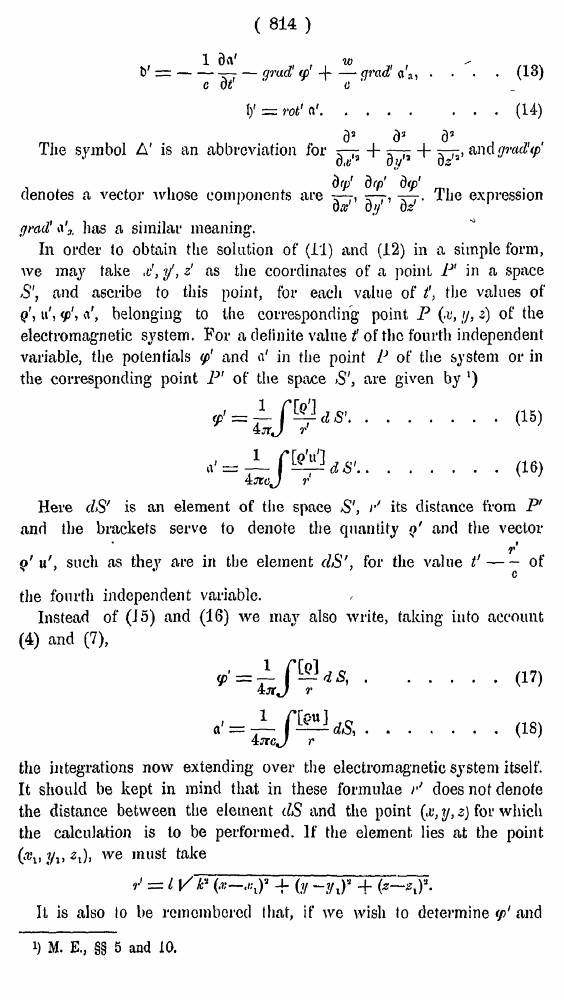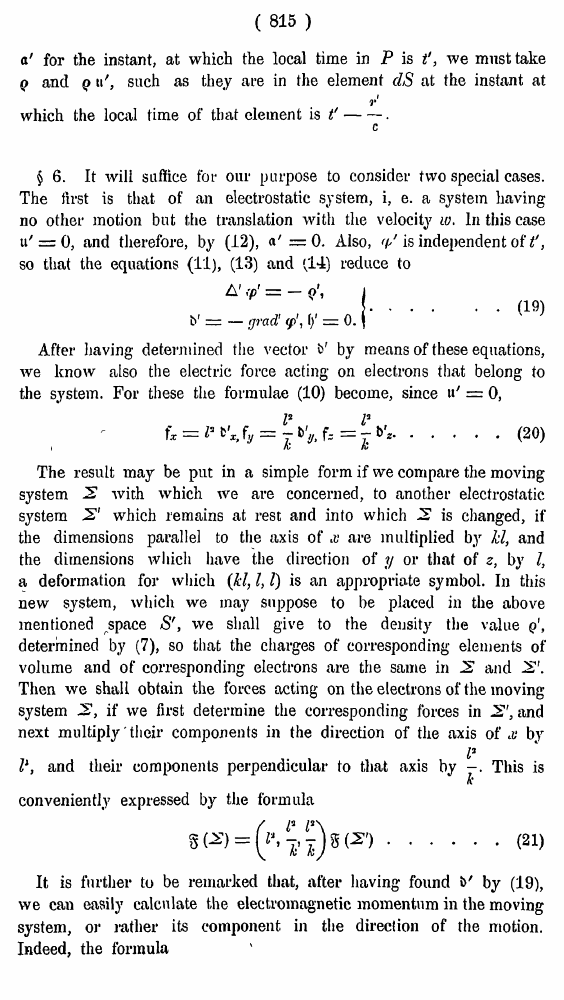# H. A. Lorentz. Electromagnetic phenomena in a system moving with any velocity smaller than that of light. // Proceedings Royal Acad., Amsterdam. Vol. VI., 1904

ô ÚÁ¼ÁŠŸ   áÞµÐÒÍ ¶ŸÞšÁ·«   <<<     î·ÞÁÚÒ—Á 814   >>>809  810  811  812  813  814 815  816  817  818  819  820  821  822  823  824  825  826  827  828  829  830  831 ( 814 ) . . . (13)    (14) The symbol A' is an abbreviation for, and gmdUp denotes a vector whose components areThe expression grail' has a similar meaning;. In order to obtain the solution of (11) and (12) in a simple form, we may take &, y, z' as the coordinates of a point P1 in a space S', and ascribe to this point, for each value of ?, the values of q', u', \$', are given by ') ......(15) ......(16) Here clS' is an element of the space S', r' its distance from P' and the brackets serve to denote the quantity ;'A> 2i)i we must take It is also lo be remembered that, if we wish to determine ' by means of these equations, we know also the electric force acting on electrons that belong to the system. For these the formulae (10) become, since u' = 0, .....(20) i The result may be put in a simple form if we compare the moving system 2 with which we are concerned, to another electrostatic system 2' which remains at rest and into which 2 is changed, if the dimensions parallel to the axis of x are multiplied by hi, and the dimensions which have the direction of y or that of z, by I, a deformation for which (kl, I, I) is an appropriate symbol. In this new system, which we may suppose to be placed in the above mentioned space S', we shall give to the density the value q, determined by (7), so that the charges of corresponding elements of volume and of corresponding electrons are the same in 2 and 2. Then we shall obtain the forces acting on the electrons of the moving system 2, if we first determine the corresponding forces in 2', and next multiply their components in the direction of the axis of ' by (19), we can easily calculate the electromagnetic momentum in the moving system, or rather its component in the direction of the motion. Indeed, the formula (21)